# Glencoe Geometry Chapter 1 Test Answers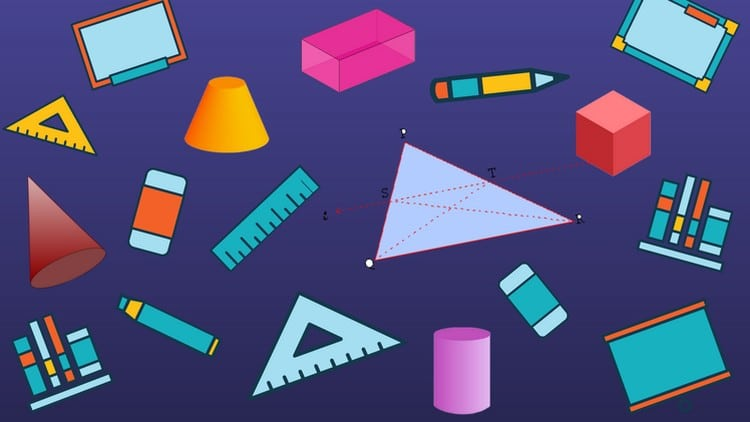We thoroughly check each answer to a question to provide you with the most correct answers. Found a mistake? Let us know about it through the REPORT button at the bottom of the page.

## Glencoe Geometry

Glencoe Geometry is a registered trademark of McGraw-Hill, which is not affiliated with Quizzma.com.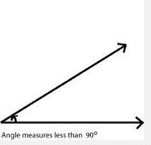acute angle
An angle that measures between 0° and 90°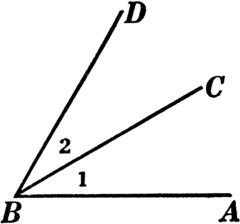two angles that have a common vertex and side but no common interior points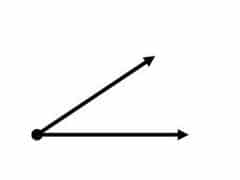angle
formed by two rays with a common endpoint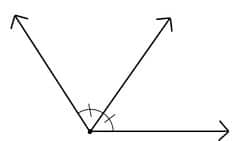angle bisector
a ray that divides an angle into two congruent angles
area
The measure, in square units, of the inside of a plane figure.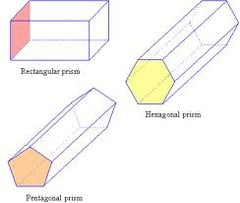base of a prism
between
When three points are collinear, you can say that one point is ____________ the other two.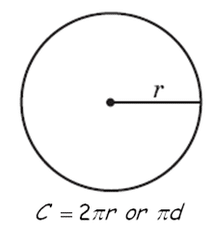circumference
The distance around a circle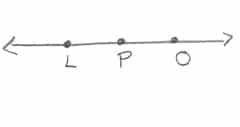collinear
on the same line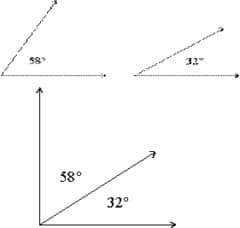complementary angles
Two angles whose sum is 90°.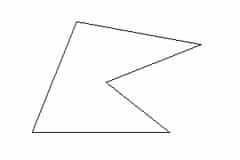concave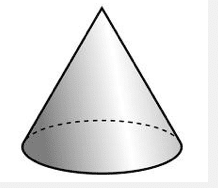cone
a solid figure with one circular base and one vertex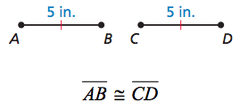congruent
Having the same size and shape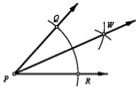construction
uses compass and straightedge, but no measuring tools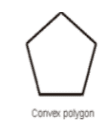convex
polygon where no points of the lines are in the interior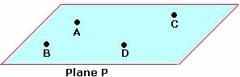coplanar
points, line, segments, rays that are on same plane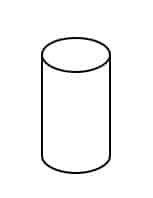cylinder
a solid with congruent parallel circular bases connected by a curved surface
degree
Unit of measurement for an angle that is 1/360 of a circle
distance
length of segment from one endpoint to the other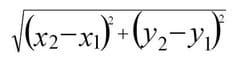distance formula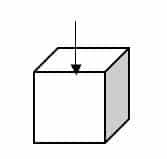edge
The line segment where two faces of a solid figure meet.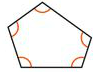equiangular polygon
polygon which all angles are congruent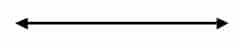line
A basic undefined term of geometry; extend indefinitely and have no thickness or width.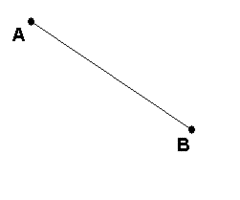line segment
two endpoints and all the points between the endpoints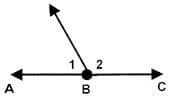linear pair
two adjacent angles with their noncommon sides forming opposite rays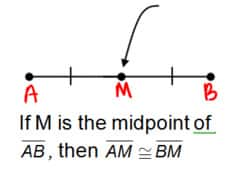midpoint
The point that divides a segment into two congruent segments.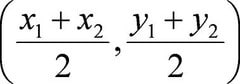midpoint formula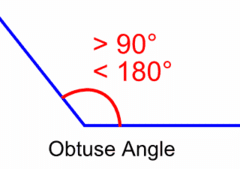obtuse angle
an angle that measures more than 90 degrees and less than 180 degrees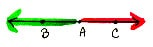opposite rays
Two rays that have a common endpoint and form a line.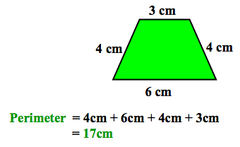perimeter
the distance around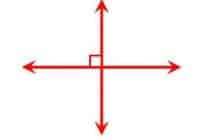perpendicular
two lines, rays, or segments that form a 90 degree angle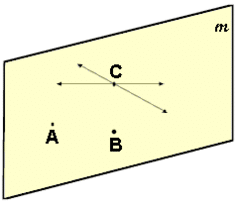plane
A flat surface that extends in all directions containing at least 3 noncollinear points.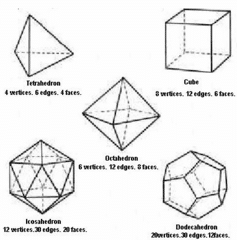platonic solid
point
a specific location in space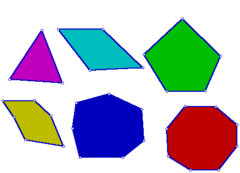polygon
a closed plane figure formed by three or more line segments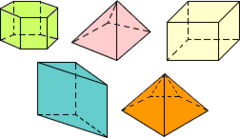polyhedron
a 3D figure whose faces are polygons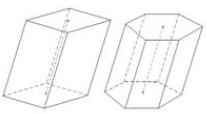prism
polyhedron with two parallel congruent faces called bases connected by parallelogram faces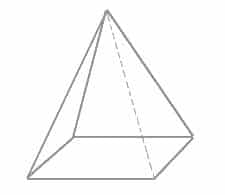pyramid
A solid figure that has triangles for its sides and a polygon as its base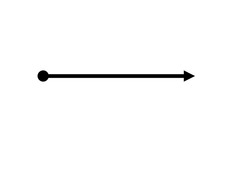ray
part of a line that has one endpoint and extends infinitely in one direction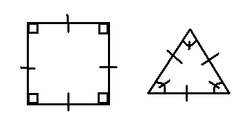regular polygon
a polygon in which all sides are congruent an all angles are congruent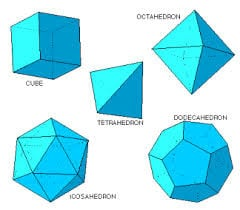regular polyhedron
all faces are regular congruent polygons. all edges are congruent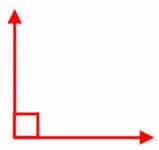right angle
An angle that is exactly 90.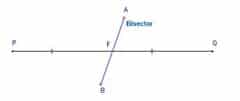segment bisector
The segment that cuts another segment at its midpoint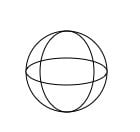sphere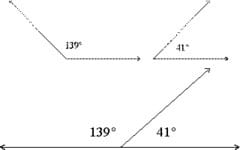supplementary angles
surface area
The sum of the areas of the faces of a geometric solid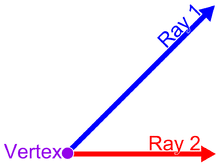vertex of an angle
point where two lines, line segments, or rays meet to form an angle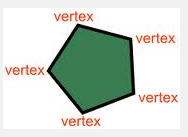vertex of a polygon
where segments on a polygon meet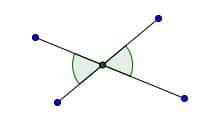vertical angles
two nonadjacent angles formed by intersecting lines or segments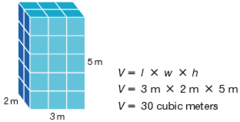volume
the amount of three-dimensional space enclosed in a geometric solid

## Glencoe Geometry Chapter 1 Vocabulary

undefined terms
point, line, and plane
point
location
line
made up of points and has no thickness or width
collinear
points on the same line
plane
a flat surface made up of points
coplanar
points on the same plane
A point is named by:
a capital letter
Line is named by:
letters representing the points or a lowercase script letter
Plane is named by:
a capital script letter or by the letters naming three noncollinear points
Space
boundless, three-dimensional set of all points
Line segment
can be measured because it has two endpoints
Midpoint
point that devides the segment into two congruent segments
segment bisector
Any segment,line, or plane that intersects a segment at its midpoint is called this:
Degree
What we measure angles with
Ray
Part of a line with one endpoint and the other end extends infinately
Opposite Rays
a point on the line has rays going in either direction
Angle
formed by two noncollinear rays that have a common endpoint
Right angle
90 degrees
Acute Angle
less than 90 degrees
Obtuse Angle
Between 90 and 180 degrees
Angle Bisector
Ray that divides an angle into two congruent angles
are a pair of angles with a common vertex and a common side, but no common interior points
angle bisector
a ray that divides an angle into two congruent angles
between
in the middle of
betweeness of points
a point is between two other points on the same line
complementary angles
Two angles whose sum is 90 degrees
concave
curving inward
congruent
Having the same size and shape
construction
drawing a figure satisfying certain conditions as part of solving a problem or proving a theorem
convex
no line that contains a side of the polygon contains a point in the interior of the polygon
exterior
the outer side or surface of something
interior
the inner or enclosed surface of something
linear pair
two adjacent angles whose noncommon sides are opposite rays
n-gon
a polygon with n sides
perimeter
The distance around a figure
perpendicular
intersecting at or forming right angles
polygon
a closed figure, all straight lines, and no intersecting lines
precision
a measure of how close a series of measurements are to one another
regular
No extended lines pass through interior
irregular
Extended lines pass through interior
relative error
the ratio of absolute error to actual measure
sides
line segments that form the polygon
supplementary angles
Two angles whose sum is 180 degrees
vertex
the point of intersection of lines or the point opposite the base of a figure
vertical angles
two angles whose sides form two pairs of opposite rays
triangle
3 sides
4 sides
pentagon
5 sides
hexagon
6 sides
heptagon
7 sides
octagon
8 sides
nonagon
9 sides
decagon
10 sides
dodecagon
12 sides

## Glencoe Geometry: Online Textbook Help

Anyone enrolled in a class using the Glencoe Geometry textbook should consider this companion course. You will learn the material faster, retain it longer and earn a better grade.